Home

# One-dimensional fibers of rigid subanalytic sets

## Extract

Let K be an algebraically closed field of any characteristic, complete with respect to the non-trivial ultrametric absolute value ∣·∣: K → ℝ+. By R denote the valuation ring of K, and by ℘ its maximal ideal. We work within the class of subanalytic sets defined in , but our results here also hold for the strongly subanalytic sets introduced in  as well as for those subanalytic sets considered in . Let XR1 be subanalytic. In , we showed that there is a decomposition of X as a union of a finite number of special sets UR1 (see below). In this note, in Theorem 1.6, we obtain a version of this result which is uniform in parameters, thereby answering a question brought to our attention by Angus Macintyre. It follows immediately from Theorem 1.6 that the theory of K in the language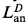(see  and ) is C-minimal in the sense of  and . The analogous uniformity result in the p-adic case was recently proved in .

Definition 1.1. (i) A disc in R1 is a set of one of the two following forms:A special set in R1 is a disc minus a finite union of discs.

(ii) R-domains uRm, and their associated rings of analytic functions,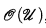, are defined inductively as follows. Rm is an R-domain and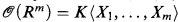, the ring of strictly convergent power series in X1,…, Xm over K. If u is an R-domain with associated ring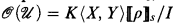, (where KX, Y〉 〚ρ〛S is a ring of separated power series, see [5, §2] and [1, §1]) and f,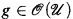have no common zero on u and ◸ ϵ {<, ≤}, then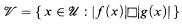is an R-domain and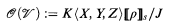where J is the ideal generated by I and fgZ (Z is a new variable) if ◸ is ≤, and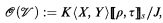where J is the ideal generated by I and fgτ (τ a new variable) if ◸ is <. (See [8, Definition 2.2].) R-domains generalize the rational domains of [2, §7.2.3]. It is true, but not easy to prove, thatonly depends on u as a point set, and is independent of the particular representation of u.

Hide All

# One-dimensional fibers of rigid subanalytic sets

## Metrics

### Full text viewsFull text views reflects the number of PDF downloads, PDFs sent to Google Drive, Dropbox and Kindle and HTML full text views.

Total number of HTML views: 0
Total number of PDF views: 0 *Loading metrics...

### Abstract viewsAbstract views reflect the number of visits to the article landing page.

Total abstract views: 0 *Loading metrics...

* Views captured on Cambridge Core between <date>. This data will be updated every 24 hours.

Usage data cannot currently be displayed.